Newsletter ListNewsletter Issue #72, Dec 2003

# SPICE Numerical Methods, Part 1 Convergence Methods

It’s been some time since we devoted a newsletter to modeling and convergence issues. Over the years, we have learned more about how to make good models and have also added and improved IsSpice options. The information shown here follows closely the seminar presentation at the Power Systems World on November 3, 2003.

Understanding what’s under the hood in your software tools has been dismissed in the quest for simple user paradigms. But your software is performing mathematical tasks that, as an engineer, you’ve been trained to understand. You’ll get the most out of our IsSpice simulation engine by combining your knowledge and experience with an understanding of how the simulator works - especially if you want to make models for other engineers to use.

 In This Issue 1 6 Unique Solution Sidebar 6 9 Spice Options for Transient Simulation Control 12 Transient Initialization 14 Making Models Behavioral Models

SPICE is basically an equation solver. The equations are set up according to Kirchhoff’s Current Law, KCL. The KCL matrix is modified to include the current through voltage sources and state variables used in the XSPICE model extensions. For linear time invariant circuits, the equation matrix has a unique solution (See the Unique Solution Sidebar). Non-linear circuits and operations involving integration require inserting a Norton equivalent circuit with the large signal current summed into the matrix’s right hand side, RHS, and the small signal conductance summed into the matrix.

This summation process is referred to as the matrix stamp of a SPICE model. Interestingly, each model sums its contributions without the knowledge of what the other models are doing. Figure 1 illustrates the matrix stamp for a diode. When the diode anode is node K and its cathode is node L, then the K row and column and the L row and column have the small signal conductance summed into their matrix positions as shown. The large signal current is summed with appropriate signs into the RHS.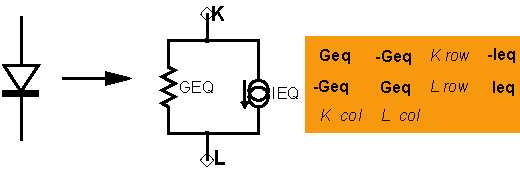Figure 1. Diode linearized circuit and its matrix stamp.

SPICE must iterate this solution to solve non-linear circuit equations. The simulator doesn’t know if the circuit is linear so that it will iterate linear circuit equations as well. Certain linear circuit equations may fail to converge if the RHS vector is not a constant. Remember, the basic KCL rule is that the sum of the currents at a node is constant. If it’s a variable, then there is no guarantee of convergence.

By way of example, consider the circuit and its modified nodal admittance, MNA, matrix shown in Figure 2. This circuit model is used to describe a transformer. Current is transformed from the left or input side to the right or output side. Voltage is transformed in the opposite direction. These equations force the output power to equal the input power. The model is useful for any power conserving operation. In the third equation, the current at B4 is .5 * IR1. It must remain on the RHS because IR1 is not a matrix variable. For the circuit configuration shown, v2 grows for each iteration as shown below:

 Iteration v2 IR1 1 0 10k 2 250k -2.499e+007 3 -6.2475e+008 6.2475e+010

Clearly, the solution is not converging; v2 is alternating sign and its magnitude is growing rapidly to infinity. If the input and output of the model were reversed, then the solution would have converged.Figure 2. An equation matrix example incorrectly using the current through a resistor.

Now consider the circuit shown in Figure 3. The input current is sensed through a voltage source instead of a resistor. The current through the voltage source is a matrix variable. The SPICE3 arbitrary source or B-element saves the variables and their derivatives and uses that information to move any RHS components using those variables back over to the matrix during the load operation as shown in the figure. With this seemingly minor change in the model, the circuit has been made to converge without the need for any iterations. The lesson learned here is to make B-element equations that only use matrix variables. That isn’t to say that you can’t write equations with variable RHS values; however, it’s unwise to rely on the solution iterations to converge.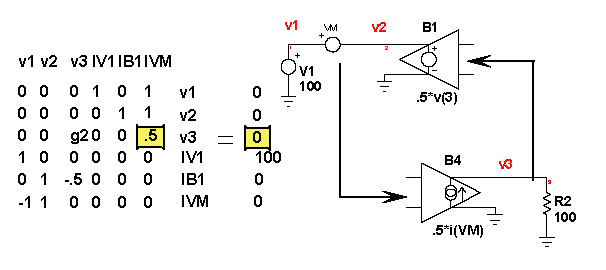Figure 3. A properly formed model allows the B-element to move the current from the RHS into the matrix.

In order to handle non-linear algebraic equations, SPICE simulators perform what is known as Newton-Raphson iteration. The linearized matrix; for example, one using a diode as shown in Figure 1, is solved and a new operating point and its small signal equivalent admittance is found. The process is repeated until a stable solution is found. Figure 4 shows a test circuit using a diode and Figure 5 shows how the equation is iterated to form a stable solution. It seems pretty reasonable to model a diode voltage as a function of current – that’s what designers usually do to estimate an operating point because a Silicon diodes forward drop ranges from .6 to .7 volts for very large range of current.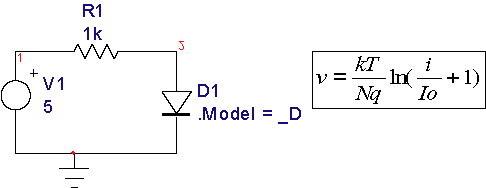Figure 4. A diode test circuit, the operating point converges in 5 iterations.Figure 5. Newton-Raphson iterations converge rapidly to solve for the operating point

However, early on, the problem of finding a stable solution for this circuit used the equations formulated with current as a function of voltage as shown in Figure 6. You can see from inspection that the initial trial would place nearly 5 volts across the diode resulting in 8.348e+069 amps. The next iteration would produce nearly the same result and it would take forever to walk the solution back to the correct answer. The funny thing is that SPICE3 quickly sees the convergence failure and tries some interesting tricks to make even this ill conditioned equation work.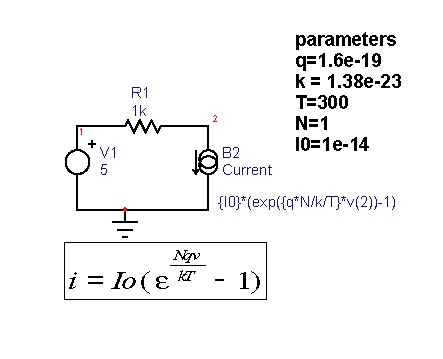Figure 6. Using a B-element with current as a function of voltage requires 194 iterations to converge

When the DC iteration limit, ITL1, is exceeded (it defaults to 100 iterations). Then the simulator tries a neat trick called Gmin Stepping and finally it tries Source Stepping. Before attempting a matrix solution, SPICE preorders the matrix by reordering its rows and columns to get large values along the matrix diagonal. If the only values in the matrix were on the diagonal, the solution can be written by inspection and is trivial. By the same argument, if the values on the matrix diagonal are very much larger than the off-diagonal values, then a solution should be readily achieved. That’s the idea behind Gmin Stepping. Gmin is a SPICE option representing the smallest possible conductance. SPICE3 begins by setting the number of steps to 10, then computes the diagonal offset as Gmin*10^numSteps. For the default Gmin=1e-12, the initial offset if .01. That corresponds to a 100 ohm resistor to ground on the diagonal nodes. If convergence fails on the first step, then the simulator signals Gmin Step failed (you might want to increase the numGminSteps or increase Gmin). Intusoft has made several modifications to these algorithms to improve their performance. You can see that a 100 ohm resistor across the diode initializes much closer to the correct solution for our example. The offset is gradually removed and if on its final removal, the circuit converges, the job is done. If not SPICE3 tries source stepping. All voltage and current sources are set to zero and stepped up to their final values using the number of steps specified using ITL6. If you set ITL1=50 for this case, then Gmin Stepping fails and the simulator will go on to use source stepping. Source stepping will walk the solution up the I-V curve from V=0 and will also converge. As you see, IsSpice works pretty hard to make even poorly constructed models converge. When you build your own models, you need to keep the accounts option turned on (.OPTIONS acct) and keep an eye on the operating point iterations. Most modern SPICE simulators employ variations of the described operating point convergence methods; however, the different vendors have fine tuned the algorithms and hold these methods as trade secrets.

Before continuing to discuss IsSpice options, it’s worth comparing the performance of the built-in diode with its behavioral counterpart shown in Figure 7. Running this simulation and checking the number of iterations, we find the solution converges in 9 iterations. That’s about twice as many iterations needed by the built in SPICE diode. The SPICE diode model is more complete (it include the temperature effects of IO, noise, …). Even so, the added complexity comes with built-in convergence aids that are hard to beat with behavioral models. We’ll visit this topic in more detail later on.Figure 7. Diode modeled using a B-element voltage source converges in 9 iterations.

TOP

 Unique Solution Sidebar

An interesting property of the linear matrix solution arises for the AC analysis. It turns out that the solution does not diverge if the circuit is unstable in the time domain. The feedback equation

G = A/(1+A*H) has the same answer as A—> infinity or A—> -infinity! - A result that allows you to analyze unstable circuits for gain and phase margins. Moreover, you can insert high gain amplifiers nearly anywhere in a circuit to force the “amplifier” input node voltage or branch current to null. You can use that technique, called null injection, to isolate a section of circuitry while a control loop is closed. Using various combinations of null injection, you can solve for A, AH, H and G in the feedback equation. This technique has been expanded to become the General Feedback Theorem, by Dr. R.D. Middlebrook .

 R.D.Middlebrook, “The GFT: A General Yet Practical Feedback Theorem,” to be published.

 Spice Options for Operating Point Control

The following are the key options that will aid in making your DC operating point converge.

1. GMIN: Sets the minimum conductance (default is 1e-12), see previous discussion with respect to its use in Gmin Stepping. It is also used within various models to eliminated divide by zero errors. Typically, divisions have Gmin added to the numerator and the denominator as follows: A/B —> (A+Gmin)/(B+Gmin). This approach yields an answer for B=0 and both A and B zero. The odds of B = -Gmin are greater than 1 in 2^48 = 2.8e+014 because the 64 bit floating-point mantissa is 48 bits long. On the other hand, the odds of having a zero value is extremely high because many variables are initialized to zero. For your B-element equations, you can use Gmin as a parameter or go ahead and hard wire a reasonable value, for example 1u, into your divisions.

2. RELTOL (default=.001), ABSTOL(default=1e-12), VNTOL(default=1e-6) are used after each Newton-Raphson iteration to decide on whether or not each node voltage or branch current is converged. If val is the value just iterated and prev is the previous value, the following logic is applied:

a. Voltage: tol = abs(val)*RELTOL+VNTOL
b. Current: tol = abs(val)*RELTOL+ABSTOL
c. If abs(prev-val) > tol return(NONCONV)

These equations appear to be reasonable for a circuit that’s working normally; however, SPICE can reach some very extreme values. As abs(val)—> infinity, the tolerance gets very large and absurd values may appear to converge. You may encounter this condition when attempting to float a section of circuitry. The floating circuit node voltages become independent of SPICE ground. The DC offset can get so large that a matrix solution becomes impossible. The solution is to not float circuitry. You may wish to use a parameterized resistor between the 2 circuit grounds to see the effect on the floating voltage vs. resistor value. Many models operate in the 1 to 10 volt range making VNTOL in the range of 10u to 100u a reasonable choice. Similarly branch currents in the 1 to 10ma range would suggest ABSTOL in the range of 1 to 10n. RELTOL is frequently set to .01. The ABSTOL and VNTOL setting apply to every node voltage and branch current so that you should scale models in a similar range. If you need to maintain accuracy for very small numbers; for example, when time delay is a variable, then you should scale your model to compute the time delay in microseconds or milliseconds as the case may be. Alternatively you can use normalizing B-elements to multiply the voltage or current such that the result is in the proper range. These tolerances are also applied when calculating the local truncation error, LTE, for time step control.

3. RSHUNT(default not used): The RSHUNT value is connected from every node to ground. This is useful in tracking down singular matrix problems that occur when ideal capacitors are connected in series. Without resistors, there is no unique solution for series capacitors. RSHUNT can also be used to converge problem circuits in order to explore problem areas. Making RSHUNT small enough should make almost anything converge, the example in Figure 2 can be made to converge by setting RSHUNT to 1m. RSHUNT should be considered a debugging tool because its use could introduce errors in other models that use high impedance circuitry such as integrators or charge amplifiers.

4. ITL1(default=100): The DC iteration limit. For complex circuits you may need to increase ITL1 up to 1000. Beyond that, you are likely to experience chaotic behavior and converge by chance. Reducing ITL1 can move more quickly to source stepping.

5. ITL6(default = 10): The number or steps used in source stepping. You may need to set this as high as 1000 for complex circuits. You might want to use .NODESET described later to get convergence to occur during the initial Newton-Raphson operating point iteration.

6. AUTOTOL(default not used): This is another debugging tool. It causes a table of VNTOL and ABSTOL values to be setup for each node voltage and branch current. Whenever a non-convergent situation arises, the table value is multiplied by abs(AUTOTOL). That process will rapidly eliminate the troublesome nodes. If AUTOTOL is negative, the activity is reported in the “.out” file. Examining the report may help isolate convergence problems.

7. .NODESET: This is not part of the .options commands; however, its use is important in steering the initial operating point solution to one of several stable solutions. Figure 8 illustrates how this works for a bi-stable circuit. In our previous newsletter, NL71-pg13, we showed how to add an additional non-linearity to eliminate the low voltage operating point. If the .NODESET works, it provides a simpler, more elegant solution.Figure 8. use .NODESET=100 for the operating point shown and .NODESET=0 for the low voltage operating point

 Spice Options for Transient Simulation Control

1. METHOD: Selects Gear or Trapezoidal integration. Gear works best for power electronics or other circuits that use inductors; especially when the inductor current is switched rapidly. SPICE diodes with reverse recovery will snap off and cause numerical artifacts if Trapezoidal integration is used as shown by Figure 9.

2. MAXORD(default=2): Selects the integration order for GearFigure 9. PWM Voltage, Vsw, converges rapidly using Gear integration

3. TRTOL(default=7): Transient error tolerance. The factor by which SPICE overestimates local truncation error, LTE. LTE is the estimate of integration error. Each component that uses the SPICE implicit integration method computes the time step required to achieve the desired accuracy. The smallest time step from these calculations is used for the next time step. If the required time step is less than the current time step or if the Newton-Raphson solution doesn’t converge or if the VSECTOL condition isn’t met, the time step is scaled back. Increasing TRTOL will increase the time step. This parameter was empirically selected to give the best performance over a wide range of circuits. It shouldn’t be changed. TRTOL is also used to make the VSECTOL options have hysteresis.

4. ITL4(default=10): Sets the lower transient iteration limit. This may need to be increased up to 100 for complex circuits. If this must be set higher to achieve convergence, then you may be experiencing chaotic behavior and small changes in initial conditions could cause non-convergence.

5. CHGTOL(default = 1e-14): Sets the maximum charge error, only used in the LTE calculation.

6. VSECTOL(default off): If VSECTOL is turned on, the value represents the maximum volt-second error for any node voltage. Node voltages for the next time step are predicted based on their history. The next time step begins with the predicted values. If the product of the time step and the absolute value of the difference between the iterated value and the predicted value is greater than VSECTOL, then the time step is scaled back. TRTOL is used to provide hysteresis in that decision. The time step won’t be increased until the VSECTOL computed time step is TRTOL times greater than the current time step. This option is necessary to get fast rise times from behavioral switching as illustrated by Figure 10 and 11. For the case shown, 278 time points were required to achieve the 10nsec resolution. Without VSECTOL, the maximum time step would have to be reduced to 10nsec, requiring 5e6 time points, which lengthen the simulation by a factor of 17986! The circuit shown in Figure 10 is a hysteresis switch that can be used to model under voltage lockout, UVLO. The VSECTOL option will cause the IsSpice4 simulator to backtrack; that is, reverse time and back up to just before the switching event occurs as shown in Figure 11. An R-C network is needed because the hysteresis is time-directionally sensitive; without the R-C network, the switch point can be incorrect.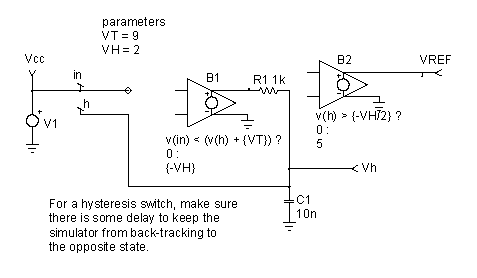Figure 10. A Behavioral hysteresis comparator shown switching with default SPICE options has a rise time equal to the SPICE time step when passing the switching threshold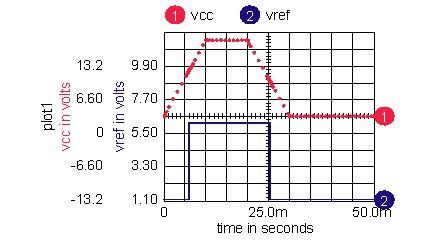Figure 11. The same simulation as Figure 10, except VSECTOL=50n made the vref signal switch from 0 to 5 volts in less than 10nsec.

7. BYPASS(default = ON): Bypasses computation of model parameters when the input voltage and currents haven’t changed very much. This speeds the simulation but can’t be used when relying on VSECTOL because large voltage and current changes may take place when rejecting time points and scaling back the time step. Turn BYPASS off when using VSECTOL.

8. RAMPTIME(default off): When set, voltage and current sources will be ramped from 0 to their specified value in RAMPTIME seconds. B-elements aren’t ramped so you can make some node voltages and branch currents non-zero. You can ramp B-elements by multiplying their output with a constant V element. This can help things like oscillators and hysteresis circuits initialize; however, the overall simulation time may be lengthened while you wait to get to steady-state. Using .NODESET is a better choice.

8. NOOPITER(default off): Skips the op calculation and goes directly to GMIN stepping

9. MINSTEP(default off): removes saved data points closer together than MINSTEP. This options reduces memory requirements for lengthy simulations; however, aliasing of data can occur.

10. MINBREAK(default=off): Breakpoints will not be set to less than this value. Breakpoints are used by various models; for example, V and I sources will set a breakpoint at the end of RAMPTIME and transmission lines will set breakpoints when a change in the propagated signal reaches the terminals. This option is most frequently used to reduce transmission line computational overhead.

11. TMAX(default off): the fourth term in the .TRAN statement can be optionally set to override the SPICE automatic time step algorithm. Frequently used in SPICE2 and SPICE3 simulators for switching power supplies. It can cause substantial increase in simulation time and memory. See use of VSECTOL to eliminate the use of this option.

TOP

 Transient Initialization

Ordinarily, the initial operating point for a transient simulation uses the DC operating point calculation. There are circuits for which this technique doesn’t work. SPICE2 and SPICE3 can direct the simulator to use initial conditions by using the UIC keyword in the .TRAN statement. When invoked, the circuit is initialized using the IC= keyword in various models and .IC v(node)=value statement. UIC works for circuits that don’t employ subcircuit models. When a subcircuit is used, the internal IC’s are used and may not correspond to the initial conditions of your external circuit. For example a positive op-amp output voltage may cause current to flow in an inductor. If you initialize the inductor current, then you must also figure out how to initialize the op-amp properly or else you might have the inductor current flowing into an open circuit. Needless to say, this is a very difficult task because you don’t know much about the internals of vendor-supplied models. Moreover, many state variables are private to models, for example diode charge, so that you can’t initialize everything. Here’s a SPICE3 script that you can use to extract the initial conditions after a transient simulation has run to steady state:

 * script to extract the final value from a transient simulation * remove everything except the node voltages set colwidth=1 nv = nextvector(null) while nv <> null len = length(nv) - 1 if len > 1 printtext -n ".IC " printname nv printtext -n "=" printval nv[len] printtext end nv = nextvector(nv) end

Just paste it into the IsSpice script window and press the DoScript button. You must remove all of the instance current and power values since they can’t be initialized using UIC. Then paste the remaining into the User Statements window of the Simulation Setup dialog. For currents, you need to find the parts and fill in the IC=value.

The most successful method for initializing complex circuits is to use switches that apply initial conditions at time = 0, and then remove the IC’s when time > 0. Like the old analog computers used to do. Figure 12 shows how it works for a simple R-L-C circuit. The IC’s were purposely set a little off to get something to look at. If you use the above script to find the IC’s, the peak error is under 7 micro-volts.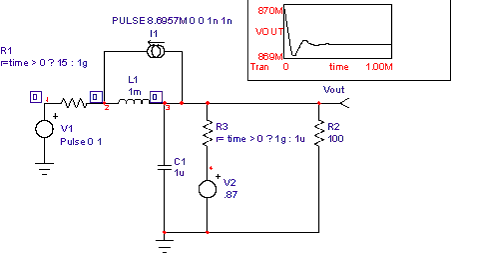Figure 12. Transient initialization without UIC.

The advantage of this technique is that SPICE will figure out the initial conditions for control circuits, so you only have to worry about the main reactive components.

 Making Models Behavioral Models

Behavioral modeling is the single most important element added to SPICE3. These B-elements allow you to introduce both linear and nonlinear models using algebraic equations. Before SPICE3 solves these equations, it removes the variable from the RHS and places them in the matrix according to the derivatives of the expression. As long as you use matrix variables (Node voltage and Voltage source branch currents) the order in which the equations are solved doesn’t matter. With SPICE3, you can pour milk into your glass BEFORE getting the glass out of the cabinet! Intusoft has added capabilities to the original SPICE3 B-elements

Intusoft B-element extensions

If-Then-Else

v=v(in) < 10 ? v(in) : 0; c/c++ style
v=MIN(v(in),10); Fortran/PSpice style

Boolean

v=(v(in) & v(uvlo)) | v(fault)
where & | ! ^ are AND, OR, NOT and XOR respectively
LONE=5, LZERO=0, LTHRESH=2.5 are default options.

Simulation Variables that can be used in expressions

TIME; simulation time in transient analysis, 0 in AC or DC
FREQ; simulation frequency in AC analysis, 0 in op or TRAN
TEMP; circuit temperature

New elements that support expressions

R=<expression>
C=<expression>
L=<expression>
Example:
R=TIME < 10u ? {OPEN} : {SHORT}

Standard B-element Operations

Math

+ - * / ^ %

Trigonometric

sin, asin, sinh, asinh cos, acos, cosh, acosh tan, atan,tanh, atanh

Transindental

exp, expl, log, lnpwr, pwrs, min, max, sgn, stp, abs, ceil, floor, int, frac, mod2, sync, mag, phs, real, imag, rand, randc

Behavioral Models – Limitations

There are some less than obvious limitations in using B-element expressions. We’ll explore these by example in the next newsletter.

We want to thank everyone who visited our booth at this year’s Power Systems World Conference. You made it an incredibly successful show for us, notably with the interest in our 8.x.10 Build 2093 ICAP/4 simulation software announced at the show, plus our collaboration with ON Semiconductor for power supply design. We look forward to some exciting product announcements for next year.

Our special half-day hands-on workshop on “Simulating and Modeling for Power Electronic Design” was a great success. All workshop attendees received a Microsoft Optical Mouse, and "Switch-Mode Power Supply SPICE Cookbook" authored by Christophe P. Basso. Again, thanks and we look forward to seeing you in 2004.

 Power Systems World Convention DRAWING WINNERS! ICAP/4Rx Power Deluxe Sofware Winner Peter Czyl - Consultant, Long Beach CA Switch-Mode Power Supply SPICE Cookbook Winners Iradj Vokhshoori - Pyramis Corp., Torrance CA Yuga Tummala - Stryker Medical, Kalamazoo MI